# Introduction-to-doremi

The main purpose of the Dynamics Of Return to Equilibrium during Multiple Inputs (doremi) package is to provide methods to estimate the dynamics parameters of self-regulated homeostatic systems experiencing multiple excitations. To do so, doremi provides functions to generate solutions of a first or second order differential equation, functions to estimate the derivatives of a given variable, and functions to estimate the coefficients of a first or second order differential equations with constant coefficients using a two-step estimation method.

In this introduction vignette, you will find in the examples section two examples of analysis that can be performed with doremi, a short presentation of what a first or second order differential equation is in the first or second order differential equation sections, and a list of the functions included in the package in the last section.

The detail of the analysis when supposing that the studied variable follows a first or second order differential equation is detailed in the associated vignettes for the first or second. The functions used to calculate the derivatives are detailed in the derivatives vignette.

# EXAMPLES

Two examples of analysis are shown below. Detailed use of the functions can be found in the vignettes for the analysis based on a first or second order differential equation. Let us consider a variable following a first order differential equation and set out of equilibrium by a given excitation function. It can be generated as follows:

# create a time vector
time <- 0:90
# create an excitation mechanism
exc <- rep(c(0,1,0),c(11,30,50))

# generate simulated data
set.seed(123)
variable <- generate.panel.1order(time = time,
excitation = exc,
tau = 5,
nind = 1,
intranoise = 1)

plot(variable)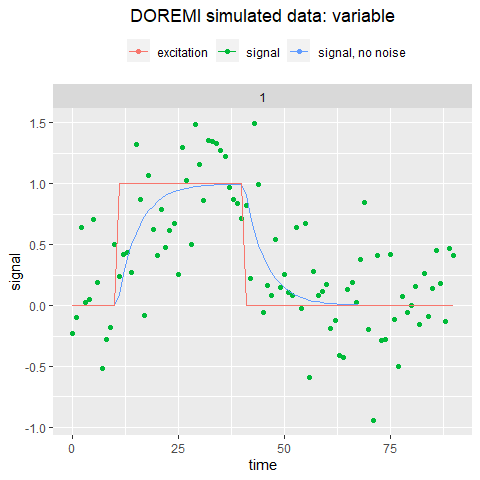The green dots are the noisy variable following a first order differential equation model that we want to analyze. The blue curve is the same without noise (i.e. the solution of the differential equation for the given parameters). The analysis can be simply performed by calling a single function:

est_result <- analyze.1order(data = variable,
input = "excitation",
time = "time",
signal = "signal",
dermethod = "gold",
derparam = 13)

The results can be plotted easily:

plot(est_result)+
ggplot2::geom_line(data = variable,aes(time,signalraw,color = "underlying model"))+
ggplot2::labs(y = "",
title = "estimation of first order\n differential equation parameters")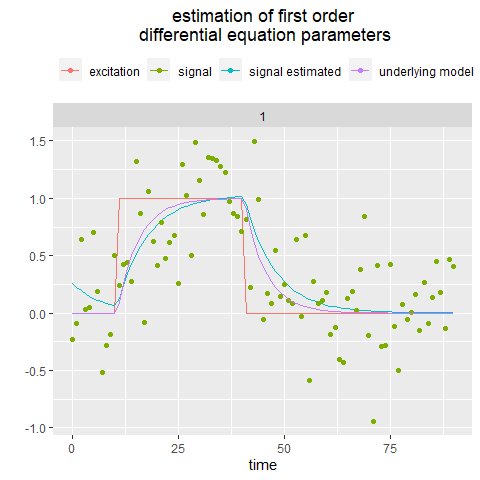The blue curve is the curve reconstructed from the coefficients estimated by the analysis, to be compared to the magenta curve, representing the model underlying the noisy data analyzed. The est_result object contains all estimated coefficients.

The same can be performed for a noisy signal following a second order differential equation driven by the same excitation. Let us generate noisy data driven by the excitation and governed by a second order differential equation:

set.seed(123)
variable2 <- generate.panel.2order(time = time,
excitation = exc,
period = 30,
y0 = 0,
xi = 0.1,
nind = 1,
intranoise = 0.8)

plot(variable2)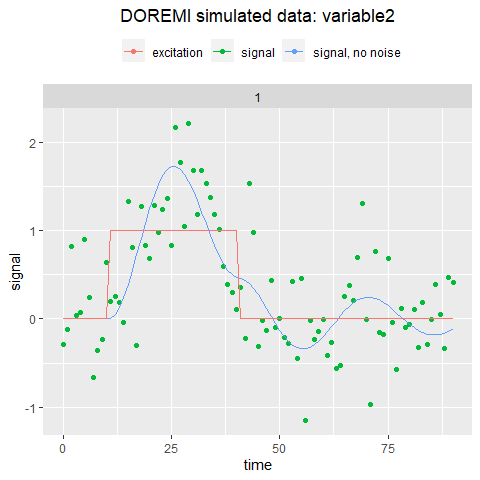We can perform the analysis based on the second order differential equation:

est_result2 <- analyze.2order(variable2,
input = "excitation",
time = "time",
signal = "signal",
dermethod = "glla",
derparam = 14)

plot(est_result2)+
ggplot2::geom_line(data = variable2,aes(time,signalraw,color = "underlying model"))+
ggplot2::labs(color = "",
y = "",
title = "estimation of second order \ndifferential equation parameters")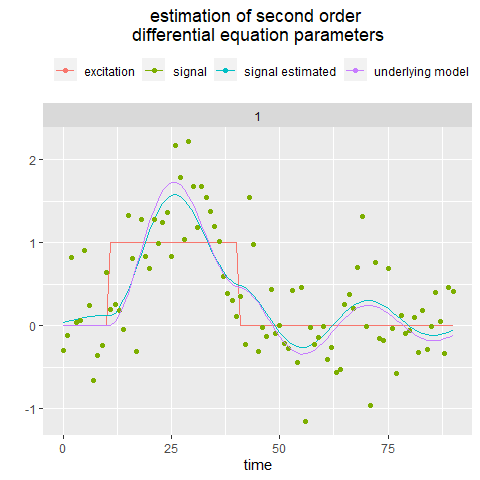The first step of the analysis consists in estimating the time derivatives of the variable. Several methods are proposed. They all depend on the number of point they consider (the embedding number) or on a smoothing parameter. More detail can be found in the section detailing the package functions and in the vignette associated. Once the derivatives are estimated, the constant coefficients of the first or second order differential equation can be estimated by a simple linear mixed-effects (multilevel) regression. As the embedding number or smoothing parameter affects the estimation of the derivatives, it will also impact the quality of the estimation of the differential equation coefficients. The package doremi provides a function optimum_param which estimates the optimum embedding number (gold/glla) or smoothing parameter (fda) by varying the latter in a range provided as input and keeping the parameter that produces the optimal estimation.

# FIRST ORDER DIFFERENTIAL EQUATION MODEL

We will shortly describe the first order differential equation and its coefficients here. A more detailed explanation of the possible analysis for a variable following a first order differential equation can be found in this vignette.

A first order differential equation with constant coefficient for the variable $$y$$ function of the variable $$t$$ (here the time) is:

$\begin{equation} \frac{1}{\gamma} \dot{y}(t) + y(t) = kU(t) + y_{eq} \label{eq1} \end{equation}$

Where:

• $$y(t)$$ is the variable to be analyzed

• $$\dot{y}(t)$$ is its first derivative, i.e. its instantaneous change

• $$U(t)$$ is the excitation term perturbing the dynamics of $$y(t)$$

The coefficients are:

• $$\gamma$$ the damping rate, the inverse of which is the damping time $$\tau = \frac{1}{\gamma}$$. The damping rate is the proportionality between the variable change and its value when the excitation term is 0. The damping time is the characteristic response time of the solution to equation (1). When the excitation starts, the variable reaches its new value and $$\tau$$ corresponds to the time needed to reach 63% of the difference between the maximum value and the initial value (it can be verified by substituting t=in the equation above). After an excitation, when the variable returns to a lower equilibrium level, the damping time corresponds to the time needed to reach ~37% (1/e) of the difference between the level reached and the equilibrium value. Figure 1 presents both the damping time $$\tau$$ for the increase and decrease of the variable.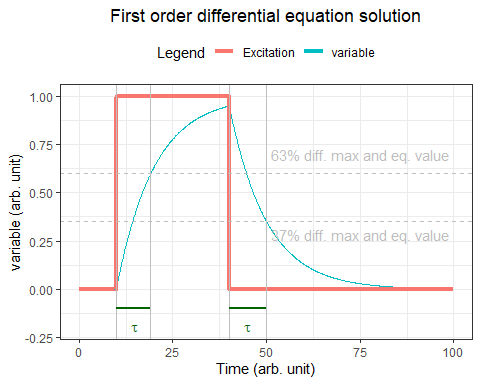• $$k$$ is the gain. It indicates the proportionality between the stationary increase of $$y$$ and the constant excitation increase that caused it.

• $$y_{eq}$$ is the equilibrium value, that is, the value the system has when there is no excitation and no remaining effect of previous excitation (i.e., when t tends to infinity).

Once the derivative estimated, doremi performs a linear mixed-effect regression to estimate these parameters. The estimated variable can be reconstructed for each individual using the numerical estimation provided by the function ode from the package deSolve.

# SECOND ORDER DIFFERENTIAL EQUATION MODEL

We will in this section shortly describe the second order differential equation and its coefficients. A more detailed explanation of the possible analysis for a variable following a second order differential equation can be found in this vignette. The differential equation considered in this case is the following:

$\begin{equation} \frac{d^2y}{dt} + 2\xi\omega_{n}\frac{dy}{dt} + \omega_{n}^2 y = \omega_{n}^2(y_{eq} + k U(t)) \label{eq3} \end{equation}$

Where:

• $$y(t)$$ is the variable to be analyzed

• $$\frac{dy}{dt}$$ is its first derivative

• $$\frac{d^2y}{dt}$$ is its second derivative

• $$U(t)$$ is the excitation term perturbing the dynamics of $$y(t)$$

And regarding the coefficients:

• $$\omega_{n} = \frac{2\pi}{period}$$ is the system’s natural frequency, the frequency with which the system would oscillate if there were no damping. The term $$\omega_{n}^2$$ represents thus the ratio between the attraction to the equilibrium and the inertia. If we considered the example of a mass attached to a spring, this term would represent the ratio of the spring constant and the object’s mass.

• $$\xi$$ is the damping ratio. It represents the friction that damps the oscillation of the system (slows the rate of change of the variable). The term $$2\xi\omega_n$$ thus represents the respective contribution of the inertia, the friction, and the attraction to the equilibrium. The value of $$\xi$$ determines the shape of the system time response, which can be: $$\xi<0$$ Unstable, oscillations of increasing magnitude $$\xi=0$$ Undamped, oscillating $$0<\xi<1$$ Underdamped or simply “damped” $$\xi=1$$ Critically damped $$\xi>1$$ Over-damped, no oscillations in the return to equilibrium

• $$k$$ is the gain. It is the proportionality between the stationary increase of the signal $$y$$ and the excitation increase that caused it. It is thus relevant only for differential equations including an excitation term.

• $$y_{eq}$$ is the variable equilibrium value, i.e. the stationary value when the excitation term is 0 or constant.

Once the derivative estimated, doremi performs a linear mixed-effect regression to estimate these parameters. The estimated variable can be reconstructed for each individual using the numerical estimation provided by the function ode from the package deSolve.

We can use generate.2order to generate a solution of this differential equation, for a given $$U(t)$$, a period of 15, and a damping factor of 0.2: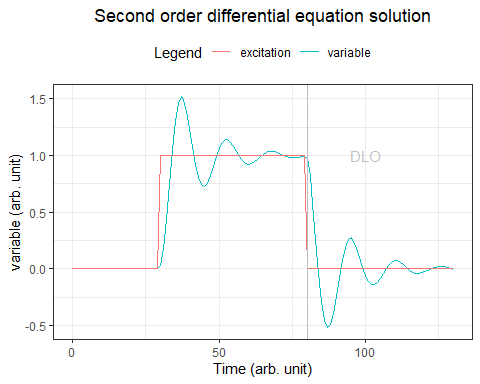# FUNCTIONS INCLUDED ON THE PACKAGE

The doremi package contains three types of functions:

### Simulation functions

These are functions that allow the user to generate data following the first/second order differential equation models (i.e. solution of equations (1)/(3)). More specifically:

• generate.1order/generate.2order: generate the solution of equation (1)/(3) with the coefficients and excitation provided as input.

• generate.panel.1order/generate.panel.2order: generate the solution of equation (1)/(3) for a given excitation and for a number of individuals, measurement noise and variability between individuals provided as input.

• generate.excitation: generates a random succession of squared pulses for a given number of points, number of pulses, amplitude and duration (used for simulation purposes only, can be used to generate the excitation variables that are introduced as input for the generate.xorder and generate.panel.xorder functions).

### Analysis functions

These functions allow to analyze a set of data and verify how close it is to being a solution of a first/second order differential equation with constant coefficients by following a two-step estimation method (derivative estimation first and then estimation of the coefficients through a multilevel regression).

• analyze.1order/analyze.2order: these functions perform estimate the derivatives of the data to be analyzed by three methods:

*GOLD- Generalized Orthogonal Local Derivative, method described in . The code available on this paper was extracted and adapted for non-constant time steps. This method allows calculating over a number of measurement points (called the embedding number) the first and second derivatives (or higher, depending on the order set as input parameter) with errors uncorrelated with the variable.

*GLLA- Generalized Local Linear Approximation, method described . This method allows to estimate the derivatives over a number of measurement points called the embedding number assuming an equally spaced time series.

*FDA- Functional Data Analysis. This method creates a function that fits the data and then derives it to evaluate the derivatives in those same points.

The analyze.1order/analyze.2order functions use one of these three methods according to what is set as input and then, once the derivatives are estimated, they are used as a known term in the multilevel regression so that the coefficients of the equation are the only unknowns and can be estimated through the fit. Once the coefficients are estimated, the estimated 1st order/2nd order variable is generated from these and the R2 is calculated and provided as a result. The estimation of the derivatives, summary of the regression, coefficients found for each individual (random coefficients of the regression) and for the group (fixed coefficients) are also provided.

• optimum_param: calculates the optimum embedding number (gold/glla) or smoothing parameter (fda) for derivative estimation by varying the latter in a range provided as input and keeping the parameter that produces the R2 the closest to 1 (optimum). It provides as output the optimum parameter found, the coefficients estimated with it and the R2 calculated.

### derivative functions

• calculate.gold: calculates the derivative of a group of data points by using the GOLD method as mentioned.

• calculate.glla: idem for the GLLA method.

• calculate.fda: idem for the FDA method.